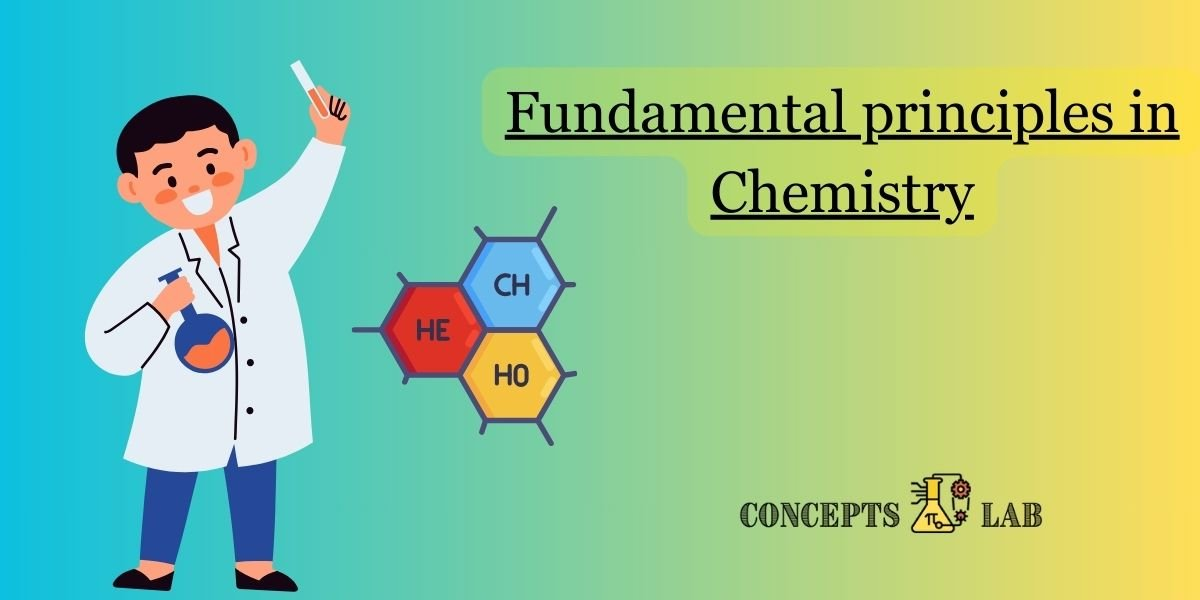Categories:

Chemistry, like physics, is built upon foundational principles that serve as the basis for understanding the behavior of matter. Here are some key first principles in chemistry:

1. Law of Conservation of Mass:
• Mass is neither created nor destroyed in a chemical reaction; it is conserved.
2. Law of Definite Proportions:
• A given compound always contains the same elements in the same proportions by mass.
3. Law of Multiple Proportions:
• When elements combine to form more than one compound, the ratios of the masses of one element that combine with a fixed mass of another element are in small whole numbers.
• Equal volumes of gases at the same temperature and pressure contain an equal number of molecules.
5. Dalton’s Atomic Theory:
• Elements are composed of atoms.
• Atoms of different elements have different properties.
• Compounds are formed by the combination of atoms in simple whole-number ratios.
• Chemical reactions involve the rearrangement of atoms.
6. Periodic Law:
• When elements are arranged in order of increasing atomic number, their properties show a periodic recurrence.
7. Bohr’s Model of the Atom:
• Electrons orbit the nucleus in fixed energy levels or shells.
• Electrons can absorb or emit energy by moving between these energy levels.
8. Lewis Dot Structures:
• Representation of the valence electrons of an atom, often used to depict the bonding in molecules.
9. Octet Rule:
• Atoms tend to gain, lose, or share electrons to achieve a full set of eight valence electrons.
10. Electronegativity:
• The ability of an atom to attract electrons in a chemical bond.
11. Mole Concept:
• The mole is a unit for counting atoms and molecules, and it is defined as the amount of substance that contains the same number of entities as there are in 12 grams of carbon-12.
12. First Law of Thermodynamics:
• Energy cannot be created or destroyed in a chemical or physical process, but it can change forms.
13. Second Law of Thermodynamics:
• The entropy (disorder) of a closed system tends to increase over time.
14. Gibbs Free Energy:
• The change in Gibbs free energy determines whether a chemical reaction is spontaneous or non-spontaneous.

These principles provide a framework for understanding the behavior of matter at the atomic and molecular levels and are foundational to the study of chemistry.